Access to comprehensive S Chand Class 10 Maths Solutions ICSE Chapter 14 Circle Ex 14(f) encourages independent learning.

## S Chand Class 10 ICSE Maths Solutions Chapter 14 Circle Ex 14(f)

Question 1.
In figure, PT is a tangent to a circle. If ∠BTA = 45° and ∠PTB = 75°, find ∠ABT.Solution:
In the figure, PQ is the tangent to the circle at T
∠BTP = 75° and ∠ATB = 45°
But ∠QTA + ∠ATB + ∠BTP = 180° (Angles of a line)
∠QTA + 45° + 75° = 180° ⇒ ∠QTA + 120° = 180°
⇒ ∠QTA = 180° – 120° = 60°
Now QTP is the tangent and TA is the chord
∴ ∠QTA = ∠ABT (Angle in the alternate segment)
⇒ ∠ABT = 60°Question 2.
In figure, TAS is a tangent to the circle, with centre O, at the point A. If ∠OBA = 32°, find the values of x and y.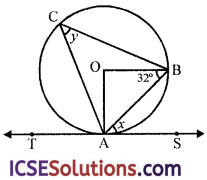Solution:
In the figure, a circle with centre O
TAS is a tangent to the circle at A
AB, AC and BC are chords of the circle
∠OBA = 32°
In △OAB,
OA = OB (radii of the same circle)
∴ ∠OAB = ∠OBA = 32°
In △AOB,
∠AOB + ∠OAB + ∠OBA = 180° (Sum of angles of a triangle)
⇒ ∠AOB + 32° + 32° = 180°
⇒ ∠AOB + 64° = 180°
⇒ ∠AOB = 180° – 64° = 116°
Now arc AB subtends ∠AOB at the centre and ∠ACB at the remaining part of the circle
∴ ∠AOB = 2 ∠ACB
⇒ 116° = 2y ⇒ y = $$\frac{116^{\circ}}{2}$$ = 58°
But ST is the tangent and AB is the chord
∴ ∠BAS = ∠ACB
⇒ x = y ⇒ x = y = 58°

Question 3.
In figure, KLMN is a cyclic quadrilateral and PQ is a tangent to the circle at K. If LN is a diameter of the circle, ∠KLN = 30° and ∠MNL = 60°, determine
(i) ∠QKN,
(ii) ∠PKL,
(iii) ∠MLK.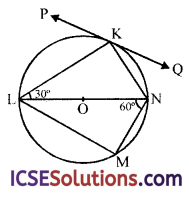Solution:
In the circle with centre O,
LN is the diameter
PQ is a tangent to the circle at K
∠KLN = 30° and ∠MNL = 60°
In △LKN,
∠LKN = 90° (Angle in a semicircle)
∠KLN = 30°
∴ ∠KNL = 90° – 30° = 60°

(i) Now PQ is tangent and KN is chord of the circle at K
∴ ∠QKN = ∠KLN = 30° (Angle in the alternate segment)

(ii) Again PQ is tangent and KL is the chord
∴ ∠PKL = ∠KNL = 60°

∠KNM + ∠KLM = 180° (sum of opposite angles)
⇒ ∠KNL + ∠LNM + ∠MLK = 180°
⇒ 60° + 60° + ∠MLK = 180°
⇒ ∠MLK + 120° = 180°
⇒ ∠MLK = 180°- 120°
⇒ ∠MLK = 60°

Question 4.
In figure, AT is a tangent to the circle. If ∠ABC = 50° and AC = BC, find ∠BAT.Solution:
In the figure, AT is the tangent to the circle and ZABC = 50°
AC = BC
∴ ∠BAC = ∠ABC = 50° (opposite of equal sides)
But ∠ACB + ∠BAC + ∠ABC = 180° (sum of angles of a triangle)
ZACB+ 50°+ 50°= 180°
⇒ ∠ACB + 100°= 180°
⇒ ∠ACB = 180° – 100° = 80°
∵ AT is the tangent and AB is chord
∠BAT = ∠ACB = 80° (Angles in the alternate segment)

Question 5.
In figure, O is the centre of the circumcircle of △XYZ. Tangents at X and Y intersects at T. Given, ∠XTY = 80° and ∠XOZ = 140°. Calculate ∠ZXY.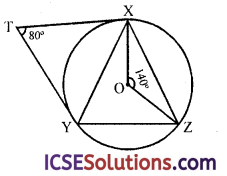Solution:
In the figure, O is the centre of circumcircle of △XYZ.
Tangents at X and Y are drawn to meet at T
∠XTY = 80° and ∠XOZ = 140°
Join OY
XT and YT are tangents to the circle
∴ ∠XTY + ∠XOY = 180° (Angles are supplementary)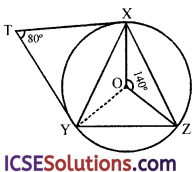⇒ 80° + ∠XOY = 180°
⇒ ∠XOY =180° – 80° = 100°
But ∠XOY + ∠YOZ + ∠ZOX = 360° (Angles at a point)
⇒ 100° +∠YOZ + 140° = 360°
⇒ ∠YOZ + 240° = 360°
∠YOZ = 360° – 240° = 120°
Now arc YZ subtends ∠YOZ at the centre and ∠ZXY at the remaining part of the circle
∴ ∠YOZ = 2 ∠ZXY
⇒ ∠ZXY = $$\frac { 1 }{ 2 }$$∠YOZ = $$\frac { 1 }{ 2 }$$ × 120° = 60°

Question 6.
In figure, O is the centre of the circle and AB is a chord of the circle. Line QBS is a tangent to the circle at B. If ∠AOB = 110°, find ∠APB and ∠ABQ.Solution:
O is the centre of the circle, AB is the chord and QBS is the tangent to the circle at B
∠AOB = 110°
Arc AB subtends ∠AOB at the centre and ∠APB at the remaining part of the circle
∴ ∠AOB = 2 ∠APB ⇒ ∠APB = $$\frac { 1 }{ 2 }$$∠AOB
⇒ ∠APB = $$\frac { 1 }{ 2 }$$ × 110° = 55°
Now QBS is the tangent and AB is the chord
∴ ∠ABQ = ∠APB = 55°
Hence ∠APB = ∠ABQ = 55°Question 7.
In figure, AB is a diameter and AC is a chord of a circle such that ∠BAC = 30°. The tangent at C intersects AB produced at D. Prove that BC = BD.Solution:
Given : In a circle with centre O and
AB is its diameter
At C, a tangent is drawn to the circle which
meet AB on producing at D
∠BAC = 30°
To prove : BC = BD
Construction : Join BC
Proof: CD is tangent and CB is chord
∴ ∠DCB = ∠BAC = 30° (Angles in the alternate segment)
In △ABC,
∠ACB = 90° (Angle in a semicircle)
∴ ∠BAC + ∠CBA = 90°
⇒ 30° + ∠CBA = 90°
⇒ ∠CBA = 90°- 30° = 60°
Now in △BCD
Ext. ∠CBA = ∠BCD + ∠BDC
⇒ 60° = 30° + ∠BDC ⇒ ∠BDC = 60° – 30° = 30°
∵ ∠BCD = ∠BDC = 30°
BC = BD (Sides opposite to equal angles)
Hence proved.

Question 8.
In figure, DE is a tangent to the circumcircle of △ABC at the vertex A such that DE || BC. Show that AB = AC.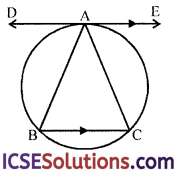Solution:
In a circle, DE is a tangent at A to the circumcircle of △ABC in which DE || BC
To prove : AB = AC
Proof : ∵ DE is the tangent and AB is the chord of the circle
∴ ∠DAB = ∠ACB ….(i) (Angles in the alternate segment)
But DE || BC
∴ ∠DAB = ∠ABC ….(ii) (Alternate angles)
From (i) and (ii)
∠ACB = ∠ABC
∴ AB=AC (Sides opposite equal angles) Hence proved.

Question 9.
In figure, two circles intersect in B and C. Lines ABD and ACE are drawn to meet the second circle at points D and E, AF is a tangent at A. Prove that AF || DE.
[Hint Join B to C]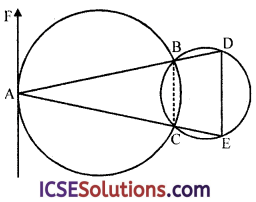Solution:
Given : Two circles intersect each other at B and C. Lines ABD and ACE are drawn to meet the smaller circle at D and E respectively. AF is the tangent to the first circle at A
To prove : AF || DE
Construction : Join BC
Proof: AF is the tangent and AB is the chord of the first circle
∴ ∠BAF = ∠ACB ….(i) (Angles in the alternate segment)
Ext. ∠ACB = Int. opp. ∠BDE ….(ii)
From (i) and (ii)
∠BAF = ∠BDE
But these are alternate angles
∴ AF || DE
Hence proved.

Question 10.
In the figure, CD is the tangent line at C to the circumcircle of △ABC intersecting AB produced in D. Show that △DBC ~ △DCA.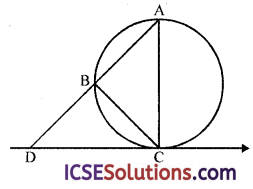Solution:
A circumcircle of △ABC, a tangent DC is drawn at C and AB is produced to meet the tangent at D
To prove : △DBC ~ △DCA
Proof: CD is tangent and BC is the chord of the circle
∴ ∠BCD = ∠BAC (Angles in the alternate segment)
Now in △DBC and △DCA,
∠D = ∠D (common)
∠BCD = ∠BAC or ∠DAC (proved)
∴ △DBC ~ △DCA
Hence proved.